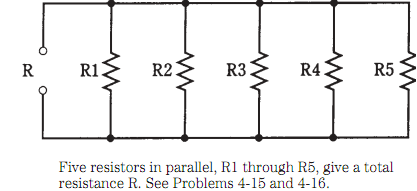## Resistances in parallel Assignment Help

Assignment Help: >> Electrical Engineering - Resistances in parallel

Resistances in parallel

When resistances are placed in the parallel, they behave differently than they do in series. Generally if you have a resistor of some value and you place other resistors in parallel with it, the overall resistance will decrease automatically.

One way to look at resistances in parallel is to take them as conductances instead. In parallel the conductances gets added, as resistances add in series. If you change all ohmic values to siemens, you can add these figures up and convert final answer back to ohms.

The symbol for conductance is G. This figure, in siemens, is related to resistance R, in ohms, by the formulas:

= 1/R, and

R = 1/G

Problem: 1

Suppose there are five resistors R1 through R5 in parallel, as shown in Figure. all having a value of 4.7K. What is the total resistance we get R?You can guess that the total is a bit less than 1K   or 1000   . So you can convert the value of the single resistor to 4,700    and divide by 5, getting a total resistance of 940. This is accurate to 2 significant figures, 9 and 4; engineers will not be worried usually about semantics, and you can simply say "940."